# Archive for the ‘Mathematics’ Category

## How to find the equation of a logarithm function from its graph?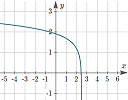This article explains how to determine the equation of a logarithmic function given its graph

## Units: Calories and kilograms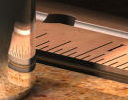How many calories? and the way the kilogram was defined has been in place since 1799. It recently got a makeover.

## Spiral around a hyperboloid - the Effekt bridge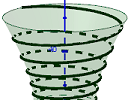We explore how to find the equations for a spiral around a hyperboloid.

## Infinite series background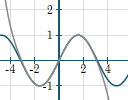Infinite series have played an important role in the development of mathematics, especially calculus. Here's some background to some applets I wrote recently.

## The object with finite volume but infinite surface area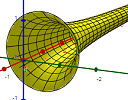This object has a weird property - it has finite volume, but infinite surface area. How is this possible?

## Double springs interactive graph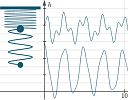The revised double spring applet now allows exploration on mobile devices. Here is some background.

## Frequency of notes on a piano - interactive learning object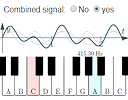Explore piano note frequencies in this interactive learning object, combining trig graphs and exponential growth.

## New applet: What does b do in a quadratic function?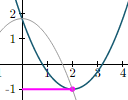y = ax2 + bx + c is a parabola. Changing the variables a and c are fairly simple, but what does b actually change, and by how much?

## New applet: Domain and range investigation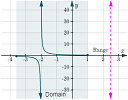This interactive applet allows you to explore the concepts of domain and range for several different mathematical functions.

## What’s the correct graph of sec(arccos(x))?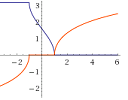Here we solve another graph mystery - what is the correct domain for y = sec(arccos(x))? And why do different software packages show similar, but different graphs?

## Full archive

See the blog sitemap for all previous articles.